/
/
/
66. At an altitude of 4 times the radius of
Not my Question
Flag Content

# Question :   66. At an altitude of 4 times the radius of : 2102146

66. At an altitude of 4 times the radius of the earth, the acceleration due to gravity is

A. g/2.

B. g/4.

C. g/16.

D. not given.

67. Suppose the gravitational potential energy for a mass at an altitude of one Earth radius is PE1 and at two Earth radii is PE2. How do the absolute values of these potential energies compare?

A.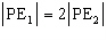B.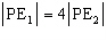C.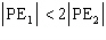D.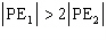but is not equal to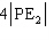68. An asteroid has a perihelion (the orbit's closest approach to the sun) of 1.5 AU and a period of revolution of 8.0 y. What is its greatest distance from the sun (its aphelion)?

A. 8.0 AU

B. 6.5 AU

C. 4.0 AU

D. 2.5 AU

69. Two satellites are monitored as they orbit the Earth; satellite X is eight times as far from the Earth's center as is satellite Y. From Kepler's third law one may conclude that the period or revolution of X is what factor times that of Y?

A. 1/2

B. 2.0

C. 4.0

D. 22.6

70. At what location does an artificial Earth satellite in elliptical orbit have its greatest speed?

A. nearest the Earth

B. farthest from the Earth

C. between Earth and Moon

D. between Earth and Sun

## Solution 5 (1 Ratings )

Solved
Physics 1 Year Ago 322 Views